# Multiplying Fractions

Learn how to multiply fractions with easeAuthor
Taylor HartleyExpert Reviewer

Published: August 24, 2023# Multiplying Fractions

Learn how to multiply fractions with easeAuthor
Taylor HartleyExpert Reviewer

Published: August 24, 2023# Multiplying Fractions

Learn how to multiply fractions with easeAuthor
Taylor HartleyExpert Reviewer

Published: August 24, 2023Key takeaways

• There are three main types of fractions — There are proper fractions, improper fractions, and mixed fractions.
• Fractions consist of a numerator and a denominator  — Both of these numbers need to be multiplied when you multiply a fraction with another fraction. However, the denominator doesn’t change when you multiply a fraction with a whole number.
• Fractions can be simplified — All you need to do is find the highest number that divides completely into both the numerator and the denominator.

The idea of multiplying fractions might seem daunting and complicated. But, the art of multiplying fractions can be mastered with just three simple steps. No more panicking before that dreaded math exam

Now, you’re probably thinking “Well, when would I actually need to multiply fractions in the real world?” The truth is, we use fractions constantly without really thinking about it. Want to work out how to split that pizza evenly between your friends? You will need to have an understanding of fractions! Looking to break that recipe in half because there’s no way you can eat 16 brownies? Similarly, you might want to triple the recipe for a school event. Both of these involve fraction multiplication.

So, how do you multiply fractions, and what steps are involved? Let’s take a closer look.

## What does it mean to multiply fractions?When multiplying whole numbers, we are adding groups of the same number.

For example:

3×4 works out as 3+3+3+3 which equals 12.

Similarly, when you multiply fractions, you are grouping fractions. The result can either be a whole number or another fraction.

For example:

½ x 3 would be ½ + ½ + ½ = 1 ½

The calculations are slightly different between problems that multiply fractions by whole numbers and those that multiply fractions together. The trick is to separate the bottom numbers and the top numbers of fractions into two different equations.

Before we dig into the steps involved in solving a fraction multiplication problem, it’s helpful to understand some of the terminology that’s commonly used:

• Numerator — The top number in a fraction
• Denominator — The bottom number in a fraction
• Whole number — Counting numbers i.e. 1, 2, 3, 4 (also known as integers)
• Factor — A number that’s being multiplied in the calculation. For example, you would multiply two factors to get the answer.
• Product — The answer to a multiplication problem

Furthermore, there are three main types of fractions that you need to be aware of:

• Proper fractions — Where the numerator of a fraction (top number) is lower than the denominator (bottom number). For example, ½ would be a proper fraction.
• Improper fractions — Where the numerator is larger than the denominator. For example, 8/3.
• Mixed fractions — The combination of a whole number and a fraction. For example, 3 ¾.

## Steps to multiplying fractionsFractions have two parts; the numerator (top number) and the denominator (bottom number). The numerators of both fractions need to be multiplied together as well as the denominators to come up with the right answer. Then, if applicable, you can simplify your answer.

### Fraction by fraction1. Multiply the numerators

Say you have a simple fraction calculation to work out. For example:

¼ x ⅔ = ?

The numerators are the numbers at the top. So, in this example, they would be 1 and 2. The first step to multiplying these fractions is to multiply both numerators. This would work out as:

1 x 2 = 2

2. Multiply the denominators

Now, you need to multiply the bottom numbers together, known as denominators. In the example above, this would work out as:

4 x 3 = 12

Then, we can put both of our answers together as their own fraction. This would be 2/12.

3. Simplify the product in the lowest form

2/12 still looks like a complicated fraction, doesn’t it? Luckily, we can simplify it! All you have to do is divide the numerator and the denominator by the greatest common factor (number) that can divide into both numbers exactly. In our example, that number would be 2. So, now we divide:

Numerator: 2 ➗ 2 = 1

Denominator: 12 ➗ 2 = 6

We can put this into a fraction: ⅙. So, now we know the answer to our original equation in its simplest form:

¼ x ⅔ = ⅙

Pretty simple, huh?

Now, let’s find out how to multiply a fraction by a whole number.

### Fraction by whole number1. Multiply the numerator with the whole number

Let’s say you have an equation that has both a whole number and a fraction. For example:

5 x ¾ = ?

Here, we need to group the fractions 5 times, which would look like this:

¾ + ¾ + ¾ + ¾ + ¾ = ?

This still looks a bit complicated, doesn’t it? Luckily, we can make the calculation simpler.

The first step is to turn your whole number into a fraction. This can easily be achieved by using your number as the numerator and adding 1 as the denominator (the denominator in any whole number is always 1). In our example above, this would be 5/1

Then, you can multiply your numerators together, just as we did above. In our example, this would be:

5 x 3 = 15

2. The denominator remains the same

We have already changed our whole number into a fraction, so we know that our calculation for the denominators is 1 x 4, which equals 4. So, basically, our denominator stays the same.

3. Simplify the product in the lowest form

So, our answer is 15/4. Now, this is an improper fraction because the numerator is larger than the denominator. There is nothing wrong with improper fractions. In fact, many mathematicians prefer to use them over mixed numbers (a whole number and a fraction). However, in the real world, we tend to use mixed numbers more often, so it’s helpful to know how to convert it.

In our example, the denominator is telling us that the whole is split into 4 equal parts, known as quarters. The numerator is telling us that we have 15 parts altogether, or 15 quarters. So, now we just need to work out how many wholes and fractions we have in their simplest form. Perhaps the easiest way to do this is with circles because they act as a great visual aid. Remember, we need to find out how many whole circles we have when we split them into quarters, and also determine if there are any fractions left over.

In our example, we have 15 parts, with each whole circle being split into quarters. With the use of a visual aid, it looks like this: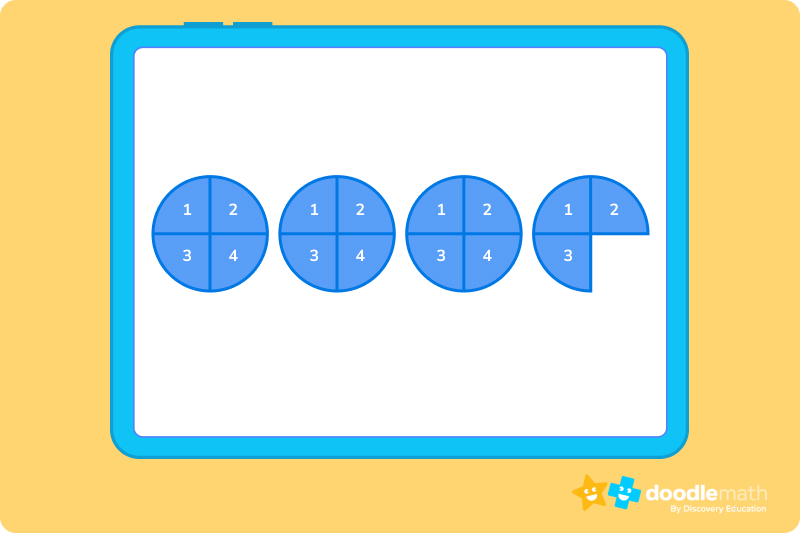Now, we have our 15 quarters. We can clearly see that we have three whole circles and three-quarters of a circle left over. This can be written as 3 ¾. We have now successfully converted our improper fraction into mixed numbers!

## Using models to represent multiplying fractionsShapes are a great way to visually represent fraction multiplication problems because they help to illustrate how the numerators and denominators interact to produce the product (answer).

You can use circles, as we did above. But, perhaps the most common shape used to represent a fraction multiplication is a rectangle because it can easily be split into equal parts.

Here’s an example:

Using a fraction model to find the product of this multiplication problem: ¼ x ⅓

1. Draw a rectangle. You need to split it into four equal columns because the denominator in the first fraction is 4. The numerator is 1. So, simply shade in one of these columns as shown below: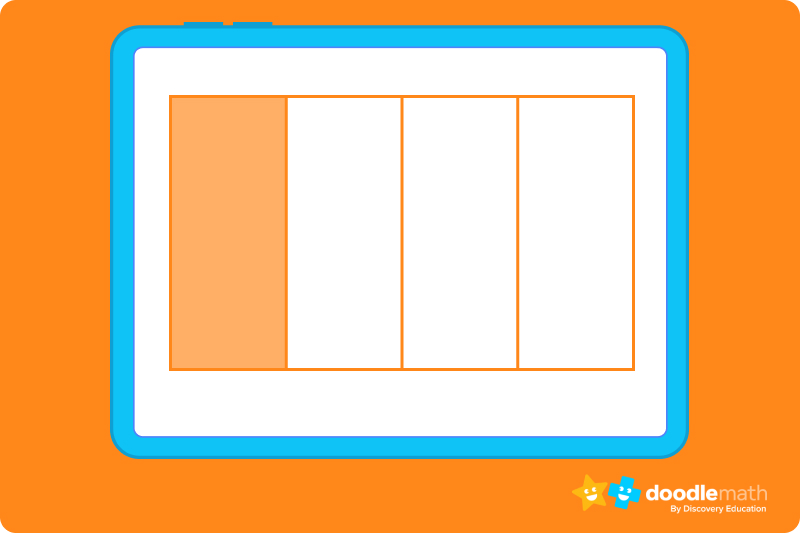2. The denominator in the second fraction is 3. So, now we add horizontal lines to represent this number: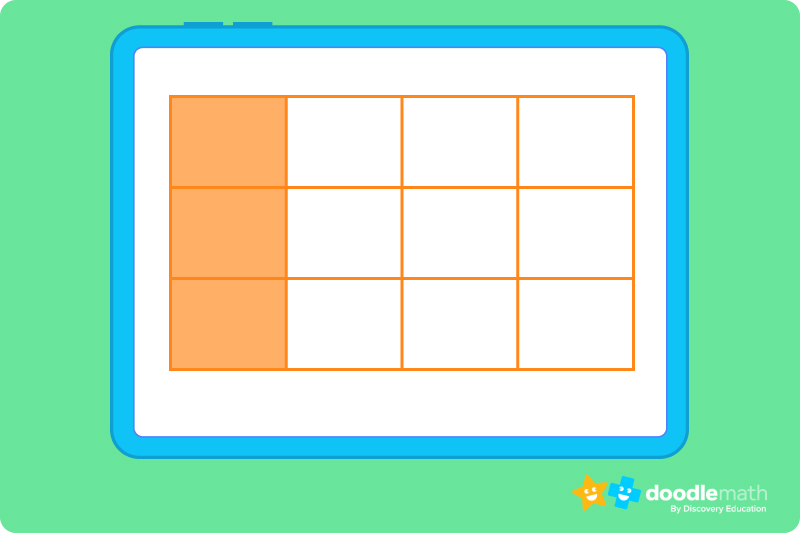3. The numerator in the second fraction is 1. So, shade in one row of your rectangle, like this: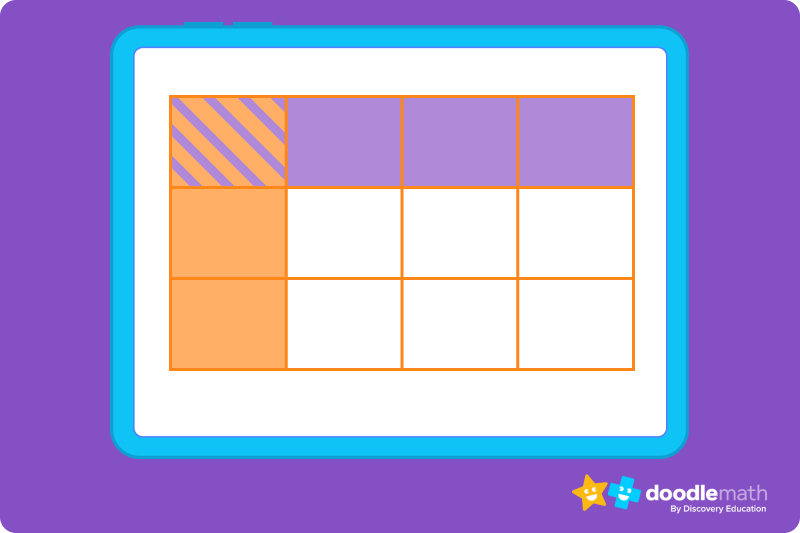4. The numerator for the answer can be worked out by simply counting the blocks that have been shaded twice. In this case, it would be 1. The denominator is the total number of blocks in our rectangle, which would be 12. So, the product of ½ x ⅓ = 1/12.

## Multiplying fractions using repeated additionMultiplying fractions with a whole number can be calculated using repeated addition. Essentially, this involves grouping fractions to determine how many whole numbers and how many leftover fractions you have. You can see this in the example above, where we used circles to work out our answer.

Let’s try another example:

Mr. Bourne gave ¼ of a cupcake to 12 of his students. How many cupcakes did he give out?

Here, we are looking to work out 12 groups of ¼. This can be written out as 12 x ¼.

¼ is also known as a quarter, so we just need to work out how many cupcakes he gave out in total. We can write it out as:

¼ + ¼ + ¼ +¼ + ¼ + ¼ + ¼ + ¼ + ¼ + ¼ + ¼ + ¼ =

But, that seems very long-winded! Instead, we can use our circle diagrams. Remember, we need to end up with 12 quarters.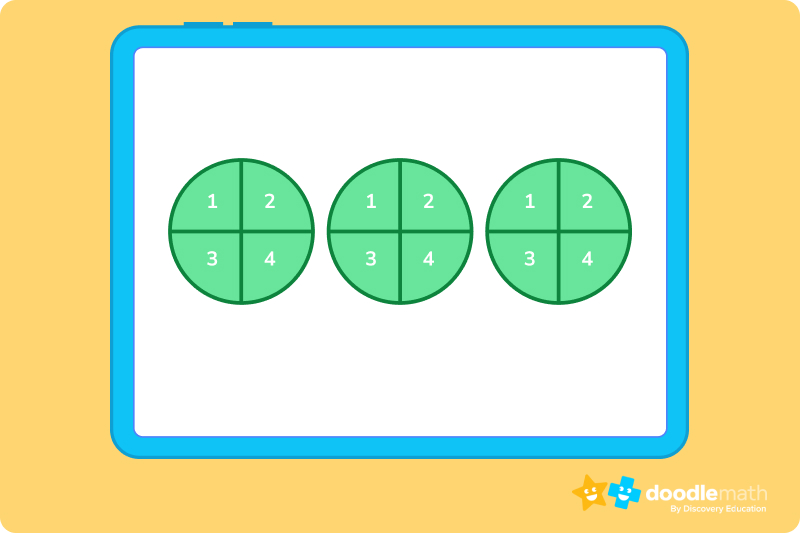From this diagram, we can see that Mr. Bourne gave out 3 whole cupcakes.

## Properties of multiplying fractionsIf you’re multiplying two fractions together, the product will be the same, no matter what order you multiply them in. For example:

¾ x ¼ will have the same product as ¼ x ¾

If a fraction is multiplied by 1, the product is the original fraction number.

If a fraction is multiplied by 0, then the product will always be 0.

When fractions are multiplied, the numerator and the denominator are multiplied separately. The numerator of the first fraction is multiplied by the numerator of the second fraction. Then, the same process is repeated with the denominators.

## Let’s practice together### 1. Solve this equation: ¾ x 2 = ?Remember that when we multiply a fraction by a whole number, we change our whole number to a simple fraction, with 1 as the denominator. This would be 2/1. Then, we simply multiply the numerators together:

3 x 2 = 6

When it comes to our denominators; 2 x 1 =  2, so the denominator in our answer remains as 2.

Therefore, the final answer to our equation is 6/4, which can be simplified to 3/2 because both numbers can be divided by 2.

### 2. Solve this equation: ⅐ x ⅗Now that we are multiplying two fractions, we already have our numerators and denominators in place. Remember, we need to treat these as two different multiplication problems:

Numerators: 1 x 3 = 3

Denominators: 7 x 5 = 35

So, we end up with 3/35. The only factor that fits into both the numerator and the denominator is 1. Therefore, this fraction is already in its simplest form

### 3. True or False: ⅔ is an improper fractionThe right answer is FALSE because the numerator is not a larger number than the denominator.

Ready to give it a go?

Now it’s time to put your knowledge to the test! Work through the following problems on your own and see how you do. Feel free to look back through this guide if you get stuck, or go through the practice problems again.

Don’t get discouraged if you struggle. Remember, practice makes perfect!

## Parent Guide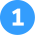How did we get here?

1. Here, we are multiplying a fraction by a whole number. So, we need to change our whole number into a fraction, with 1 as the denominator. We can then multiply the numerators together; 2 x 3 = 6
2. The denominator stays the same because 3 x 1 = 3. Now, we have 6/3 as our product.
3. We can simplify this product to 2/1 because both numbers can be divided equally by a factor of 2.
4. 2/1 is telling us that we have 2 wholes. Any numerator which has a denominator of 1, is a whole number. So, in its most basic form, our answer is just 2.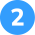How did we get here?

1. When multiplying two fractions together, we first multiply the numerators of each fraction. 4 x 3 = 12
2. Now, we multiply the denominators of each fraction. 5 x 4 = 20. This gives us 12/20.
3. This can now be simplified. The highest factor that can be divided into both 12 and 20 is 4. So we divide both numbers by 4; 12 ÷ 4 = 3 and 20 ÷ 4 = 5. Our final answer is ⅗.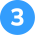How did we get here?

1. The highest factor that can be divided equally by 6 and 24 is 6.
2. 6 ÷ 6 = 1 and 24 ÷ 6 = 4. Therefore, our answer is ¼, or one-quarter.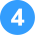How did we get here?

1. In a proper fraction, the numerator (top number) is smaller than the denominator (bottom number). So, ¾ is a proper fraction.
2. For it to be an improper fraction, the numerator would need to be a larger number than the denominator.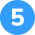How did we get here?

1. To multiply two fractions we have to multiply the numerators together: 1 x 3 = 3
2. Then, we multiply the denominators: 2 x 4 = 8
3. Don’t forget to use a visual model if you’re struggling. For example:1. Our first fraction is ½. The denominator in this example is 2, so we need 2 columns but only one of them needs to be colored in because the numerator is 1.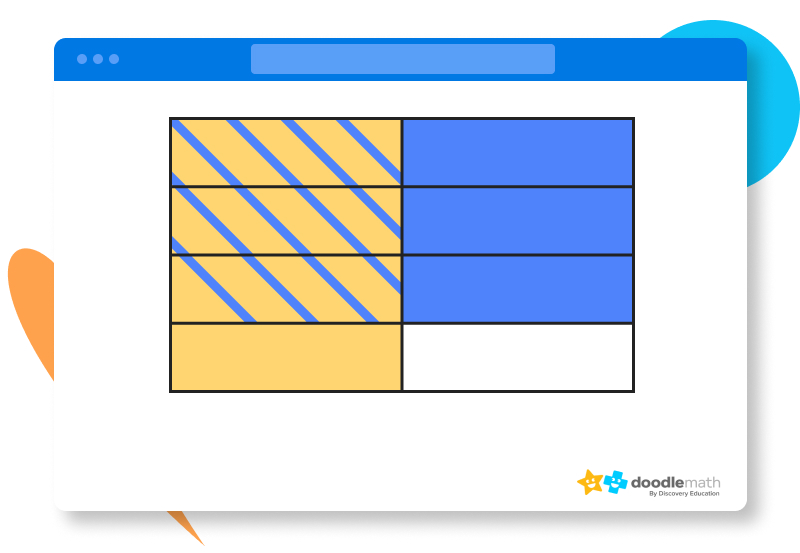1. The denominator in the second fraction is 4. So, we need 4 rows. However, the numerator is 3 which means we need to shade in 3 of the 4 rows.
2. The numerator for our product can be worked out by counting the blocks that are shaded twice. This would be 3. The denominator is the number of blocks altogether, which is 8. Therefore, the product of ½ x ¾ = ⅜.

## FAQs about math strategies for kidsWe understand that diving into new information can sometimes be overwhelming, and questions often arise. That’s why we’ve meticulously crafted these FAQs, based on real questions from students and parents. We’ve got you covered!

Like everything, practice makes perfect and it might take a little bit of time before you master multiplying fractions. However, when you break it down into simple steps, it will be much easier to understand.

Fraction multiplication can be achieved in three simple steps:

1. Multiply the numerators (top numbers) of both fractions together.
2. Then multiply the denominators (bottom numbers) of both fractions.
3. You can then simplify your answer by finding the highest factor that is divisible by both your resulting numerator and denominator.

Equations that involve a whole number and a fraction are known as mixed fractions. For example, 7 x ½. This essentially means that you have to find the product of ½ + ½ + ½. + ½ + ½ + ½ +½. To do this, change your whole number into a fraction, with 1 as the denominator. In our example, this would be 7/1. Now, you can multiply your numerators and then your denominators to find your answer.

For more complicated mixed fraction equations, you can also consider using visual models and dividing them into fractions — just like you would cut a whole pizza!

If you are multiplying a fraction with another fraction, then you need to multiply the numerators together (top numbers) and the denominators together (bottom numbers). However, if you are multiplying a fraction with a whole number then the denominator will appear to stay the same since the denominator of any whole number is 1.## Related Posts

Title 1

Title 2

Title 3

Lesson credits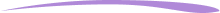Taylor Hartley

Taylor Hartley is an author and an English teacher based in Charlotte, North Carolina. When she's not writing, you can find her on the rowing machine or lost in a good novel.

Jill Padfield has 7 years of experience teaching high school mathematics, ranging from Alegra 1 to AP Calculas. She is currently working as a Business Analyst, working to improve services for Veterans while earning a masters degree in business administration.

Taylor Hartley

Taylor Hartley is an author and an English teacher based in Charlotte, North Carolina. When she's not writing, you can find her on the rowing machine or lost in a good novel.

Jill Padfield has 7 years of experience teaching high school mathematics, ranging from Alegra 1 to AP Calculas. She is currently working as a Business Analyst, working to improve services for Veterans while earning a masters degree in business administration.# Are you a parent, teacher or student?

Are you a parent or teacher?

## Hi there!

Book a chat with our team